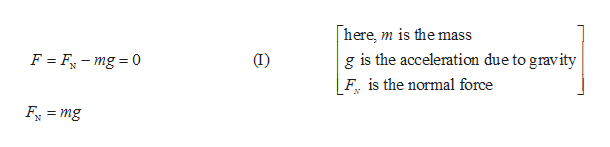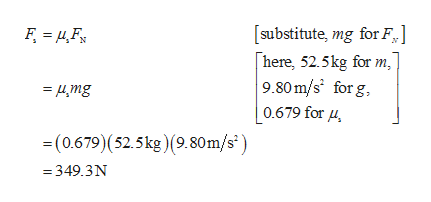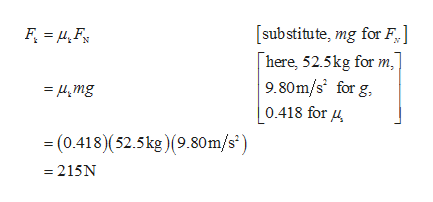A 52.5-kg crate rests on a level floor at a shipping dock. The coefficients of static and kinetic friction are 0.679 and 0.418, respectively. What horizontal pushing force is required to (a)just start the crate moving and (b) slide the crate across the dock at a constant speed?

Question

A 52.5-kg crate rests on a level floor at a shipping dock. The coefficients of static and kinetic friction are 0.679 and 0.418, respectively. What horizontal pushing force is required to (a)just start the crate moving and (b) slide the crate across the dock at a constant speed?

Step 1

The mass of the crate = 52.5 kg.

The coefficient of static friction = 0.679.

The coefficient of kinetic friction=0.418

(a)

Since the crate rests on level surface there are two forces acting on crate. One is normal force acting upwards, and other is the weight of the crate which is acting downwards.

Write the expression for force acting on cratehelp_outlineImage Transcriptionclosehere is the mass F F-mg 0 T) g is the acceleration due to gravity F is the normal force Fmg fullscreen
Step 2

The horizontal force needed to just start the crate moving is the static force.

Write the expression for kinetic force.help_outlineImage Transcriptionclose[substitute, mg for F] here, 52.5kg for m, 9.80m/s for g 0.679 for u =4mg -(0.679)(52.5kg)(9.80m/s) =349.3N fullscreen
Step 3

(b)The horizontal force required to slide the crate is the kinetic for...help_outlineImage Transcriptionclose[substitute, mg for F here, 52.5kg for m 9.80m/s for g =4mg 0.418 for 4 (0.413)(52.5kg) (9.80m/s = 215N fullscreen

Want to see the full answer?

See Solution

Want to see this answer and more?

Our solutions are written by experts, many with advanced degrees, and available 24/7

See Solution
Tagged in

Physics